## ML Aggarwal Class 7 Solutions for ICSE Maths Chapter 16 Perimeter and Area Ex 16.2

Question 1.
Find the area of each of the following parallelogram:Solution:
(i) Base of the parallelogram (b) = 8 cm and height (h) = 4.5 cm
Area = b × h = 8 × 4.5 = 36 cm2
(ii) Base of the parallelogram (b) = 2 cm and height (h) = 4.4 cm
Area = b × h = 2 × 4.4 = 8.8 cm2
(iii) Base of the parallelogram (b) = 2.5 cm and height (h) = 3.5 cm
Area = b × h = 2.5 × 3.5 = 8.75 cm2

Question 2.
Find the area of each of the following triangles:Solution:
(i) Base of the triangle (b) = 6.4 cm and height (b) = 6 cm
Area = $$\frac { 1 }{ 2 }$$ × b × h
= $$\frac { 1 }{ 2 }$$ × 6.4 × 6 cm2
= 19.2 cm2
(ii) Base of triangle (b) = 5 cm and height (h) = 6 cm
Area = $$\frac { 1 }{ 2 }$$ × b × h
= $$\frac { 1 }{ 2 }$$ × 5 × 6 = 15 cm2
(iii) Base of the triangle (b) = 4.5 cm and altitude (h) = 6 cm
Area = $$\frac { 1 }{ 2 }$$ × b × h
= $$\frac { 1 }{ 2 }$$ × 4.5 × 6 cm2
= 13.5 cm2

Question 3.
Find the missing values:Solution:
Area of ||gm = b × h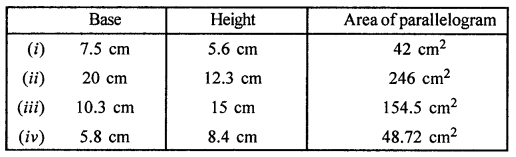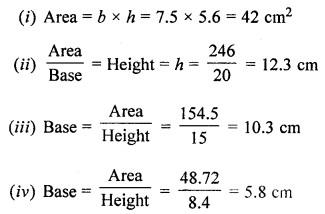Question 4.
Find the missing values:Solution: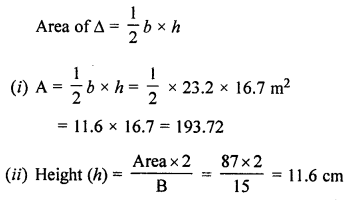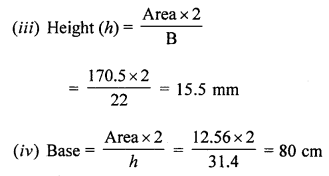Question 5.
In the given figure, ABCD is a parallelogram whose two adjacent sides are 6 cm and 4 cm. If the height corresponding to the base AB is 3 cm, find:
(i) the area of parallelogram ABCD
(ii) the height corresponding to the base AD.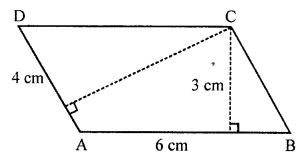Solution:
In ||gm ABCD,
Base AB (b) = 6 cm
Altitude (h) = 3 cm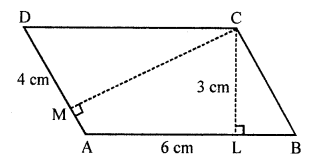(i) Area = b × h = 6 × 3 = 18 cm2
In second case,
(ii) Base = 4 cm
Area= 18 cm2
Altitude (to AD) = $$\frac { Area }{ Base }$$
= $$\frac { 18 }{ 4 }$$ cm
= 4.5 cm

Question 6.
In the given figure, ABC is an isosceles triangle with AB = AC = 7.5 cm and BC = 9 cm. If the height AD from A to BC is 6 cm, find:
(z) the area of ∆ABC
(ii) the height CE from C to AB.Solution:
In an isosceles ∆ABC
AB = AC = 7.5 cm, BC = 9 cm
Height AD to BC = 6 cm
(i) Area of ∆ABC = $$\frac { 1 }{ 2 }$$ × Base × Height
= $$\frac { 1 }{ 2 }$$ × BC × AD
= $$\frac { 1 }{ 2 }$$ × 9 × 6 = 27 cm2
(ii) Area of ∆ABC = 27 cm2
Base AB = 7.5 cm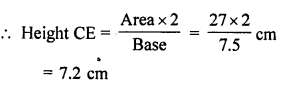Question 7.
If the base of a right-angled triangle is 8 cm and the hypotenuse is 17 cm, find its area.
Solution:
Base of a right angled triangle = 8 cm
and hypotenuse = 17 cm
Height2 = (Hypotenuse)2 – (Base)2 = 172 – 82 = 289 – 64 = 225 = (15)2
Height = 15 cm
Now are of ∆ = $$\frac { 1 }{ 2 }$$ × Base × Height
= $$\frac { 1 }{ 2 }$$ × 8 × 15 cm2 = 60 cm2

Question 8.
In the given figure, ∆ABC is right-angled at B. Its legs are 8 cm and 6 cm. Find the length of perpendicular BN on the side AC.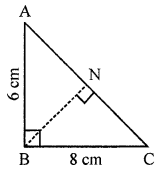Solution:
In the given figure,
In ∆ABC,
Base BC = 8 cm
and height AB = 6 cm
Area = $$\frac { 1 }{ 2 }$$ × Base × Height
= $$\frac { 1 }{ 2 }$$ × 8 x 6
= 24 cm2
Now, BN ⊥ AC
AC2 = AB2 + BC2 = 62 + 82 = 36 + 64 = 100 = (10)2
AC = 10 cm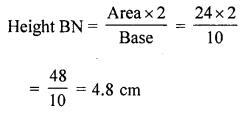Question 9.
In the given figure, the area of ∆ABE is equal to the area of parallelogram ABCD. If altitude EF is 16 cm long, find the length of the altitude of the parallelogram to the base AB of length 10 cm. What is the area of ∆AMD, where M is mid-point of side DC?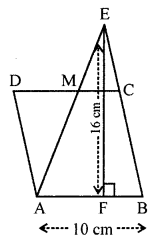Solution:
In the given figure, M is mid-point of DC
Area of ∆ABE = Area of ||gm ABCD
Now base of ∆ABC = 10 cm and height = 16 cm
Area = $$\frac { 1 }{ 2 }$$ × Base × Height
= $$\frac { 1 }{ 2 }$$ × 10 × 16 = 80 cm2
Now area of ||gm = Area of ∆ = 80 cm2
Base = 10 cm
Length of altitude = $$\frac { Area }{ Base }$$ = $$\frac { 80 }{ 10 }$$ = 8 cm
and Area of ∆AMD = $$\frac { 1 }{ 2 }$$ × Base MD × Altitude
= $$\frac { 1 }{ 2 }$$ × $$\frac { 10 }{ 2 }$$ × $$\frac { 16 }{ 2 }$$
= 20 cm2

Question 10.
In the given figure, ABCD is a rectangle of size 18 cm by 10 cm. In ABEC, ∠E = 90° and EC = 8 cm. Find the area of the shaded region.Solution:
In the figure,
ABCD is a rectangle in which
Base (b) = 18 cm
Height (h) = 10 cm
A ∆DEC is cut in which
∠E = 90°, EC = 8 cm
Now area of rectangle = l × b = 18 × 10 = 180 cm2
In ∆EBC,
BC = 10 cm, EC = 8 cm
EB2 = BC2 – EC2 (Pythagoras Theorem)
= 102 – 82 = 100 – 64 = 36 = (6)2
EB = 6 cm
Now area of right ∆EBC = $$\frac { 1 }{ 2 }$$ × EB × EC
= $$\frac { 1 }{ 2 }$$ × 6 × 8 = 24 cm2
Area of shaded portion = 180 – 24 = 156 cm2

Question 11.
In the following figures, find the area of the shaded regions: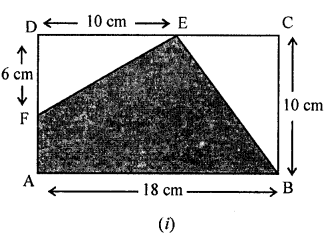Solution:
(i) ABCD is a rectangle in which
Length (l) = 18 cm
Area of rectangle = l × b = 18 × 10 = 180 cm2
DE = 10 cm
EC = 18 – 10 = 8 cm
Now area of ∆BCE = $$\frac { 1 }{ 2 }$$ × BC × EC
= $$\frac { 1 }{ 2 }$$ × 10 × 8
= 40 cm2
and area of ∆FDE = $$\frac { 1 }{ 2 }$$ × DC × DF
= $$\frac { 1 }{ 2 }$$ × 10 × 6 = 30 cm2
Area of shaded portion = Area of rectangle – Area of ∆BCE – Area of ∆FDE
= 180 – (40+ 30)
= 180 – 70
= 110 cm2
(ii) In the given figure,
ABCD is a square whose each side = 20 cm
E and F are mid-points of AB and AD respectively
EC and FC are joined
Area of square ABCD = (Side)2 = (20)2 = 400 cm2
Area of ∆EBC = $$\frac { 1 }{ 2 }$$ × EB × BC
= $$\frac { 1 }{ 2 }$$ × 10 × 20 = 100 cm2
Area of ∆FDC = $$\frac { 1 }{ 2 }$$ × FD × DC
= $$\frac { 1 }{ 2 }$$ × 10 × 20 = 100 cm2
Area of ∆AEF = $$\frac { 1 }{ 2 }$$ × 10 × 10 = 50 cm2
Area of shaded portion = 400 – (100 + 100 + 50) cm2 = 400 – 250 = 150 cm2